×
Get Full Access to The U - CHEM 1210 - Study Guide - Final
Get Full Access to The U - CHEM 1210 - Study Guide - Final

×

THE U / Chemistry / CHEM 1210 / How are ions charged?

# How are ions charged? Description

##### Description: Everything pertaining to this course formed from a combination of personal notes, madra lessons, and review topics given by the professors. Hope this helps everyone as much as possible!
18 Pages 172 Views 3 Unlocks
Reviews

Atwood, Statler Chem 1210 Fall 2017

## How are ions charged?FINAL EXAM STUDY GUIDE – GEN CHEM I

Exam 1 Material

• Unit conversions

o Common ones to know:

▪ 1 in = 2.54 cm

▪ 1 cm3 = 1 mL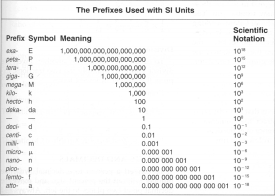▪ 1 kg = 2.2 lbs

▪ 1 ton = 2000 lbs

▪ 1 metric ton = 1000 kg

▪ METRIC PREFIXES

## What makes theory different from law?▪ Know how to read/write scientific notation

▪ Conversion factors- ratios relating two different units that can be used in  calculations **remember to raise to the same exponent when using in a  problem (ex: (1 m)3 = (100 cm)3 )

o To set up/solve: make sure that the same units are opposite from each other so  they cancel out when entire thing is multiplied

▪ Ex: 12.01 g x 1 mole x 6.022x1023 molecules

12.01 g       1 mole

• Significant Figures: Rules

## Who conducted the cathode ray experiment?If you want to learn more check out What are the steps in the mediation process?
We also discuss several other topics like What came out of the primordial soup experiment ?

o All non-zero numbers count as sig figs.

o Zeros in between non-zero numbers count

o Trailing zeros do not count unless there is a decimal point

o Multiplication/division: take answer to the same number of sig figs as the lowest  number of sig figs given in the initial problem

o Addition/subtraction: take answer to the same number of decimal places as the  lowest number of decimal places given in the initial problem

• Protons, Neutrons, and Electrons

o Protons- positively charged, make up center of the atom

o Neutrons- no charge, add mass to the center of the atom

o Electrons- negatively charged, make up the cloud around the outside of the  o Atomic number- identifies which element it is, signified by the number of  protons

Atwood, Statler Chem 1210 Fall 2017

o Atomic weight= number of protons + number of neutrons, can vary if the  element is an isotope (has a different number of neutrons)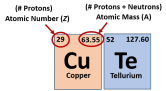We also discuss several other topics like When does lateral oppression occur?

o Charge- located in top right corner when writing element, usually lines up with  which column the element is in on the periodic table

o All atoms inherently neutral- # of protons = # of electrons

o Ions: charged versions of atoms due to a different number of electrons ▪ Cation- positive, less electrons

▪ Anion- negative, more electrons

o Isotopes: atoms with a different number of neutrons which results in a change of  atomic mass

▪ Percent relative abundance: how frequently an isotope naturally occurs ▪ Atomic mass: weighted average of all natural isotopes of an element

• Scientific Method Don't forget about the age old question of What happens to independent variables in a control group??

o Definition: the process to identify, investigate, and solve problems

o Qualitative observations: descriptive in nature

o Quantitative observations: numbers and measurements

o Hypothesis: educated guess, cause of initial observation

o Experimentation: tests, involves variables and data collection

o Law vs. Theory

▪ Law- conceptual/mathematical statement about what is observed in  nature that has been proven true

▪ Theory- neither proven nor disproven, can link laws to suggest an

underlying theme or principle Don't forget about the age old question of What are the distinctive feature of synapamorphies?

• Atomic Theory

o John Dalton (1808) stated that:

▪ Matter is made up of atoms, they are tiny, indivisible particles

▪ All atoms of the same element are identical

▪ Atoms combine in small, whole number ratios to form compounds

▪ Atoms cannot be created nor destroyed

o Law of Conservation of Matter- matter can neither be created nor destroyed,  matter in the universe is fixed and undergoes transformations

▪ Law of Conservation of Mass-Energy (Einstein)- total mass and energy in  the universe is conserved and they can interconvert

o Law of Multiple Proportions- when one element combines with another to form  a compound, the ration of masses of one element to a fixed amount of the  second is a ratio of small, whole numbersWe also discuss several other topics like What determines the equilibrium rate of interest?

Atwood, Statler Chem 1210 Fall 2017

o Law of Definite Proportions (Constant Composition)- the same compound will be  comprised of constituent elements in the same proportion by mass regardless of  the size of the sample

o J.J. Thompson

▪ Conducted the cathode ray experiment, cathode ray tube between two  charged plates

▪ Ray was attracted to the positive plate, indicated the particles were

negative

▪ Plum Pudding model of the atom: atom is a sphere of a positive charge,  with uniformly embedded negative particles throughout

o Ernest Rutherford

▪ Conducted gold foil experiment, shot alpha particles (positive charge)  through extremely thin piece of gold foil

▪ If plum pudding model was correct (expected), particles would pass

straight through the metal or be slightly deflected

▪ Instead, most of the particles passed straight through, but some were  significantly deflected

▪ Conclusion: particles deflected because of a positive, highly massive

nucleus located in the center of the atom with mostly empty space

remaining

o Robert Millikan

▪ Conducted oil drop experiment,

▪ Determined the charge of an electron to be 1.60x10-19 C

• Mole Conversions

o Amu (atomic mass units)- 1/12th the mass of a C atom, used to measure the  weight of 1 mole of a substance, same as molar mass

▪ 1 mole of any substance contains exactly this many units of the substance  (i.e. molecules, formula units)

o Molar mass- # of grams of a substance per 1 mole, located on the periodic table,  add up all the molar masses of elements in a compound to find it’s molar mass o Set up and solve the same way as for unit conversions

▪ g/mol

▪ molecules/mol

▪ mol element/1 mol compound

• Atomic Spectrum

o Bohr Model of the Atom

▪ Angular momentum of electrons and their radii were quantized, travel in  fixed orbits, and each orbit has energy associated with it

o Electron transitions

▪ Each increasing level has a lower distance between it and the previous  one (first energy jump is the largest, then get progressively smaller)

▪ The larger the distance, the more energy it requires to transition7

Atwood, Statler Chem 1210 Fall 2017

▪ ΔEn = -2.178x1018 (1/n2f – 1/n2i)

• Light

o Electromagnetic Spectrum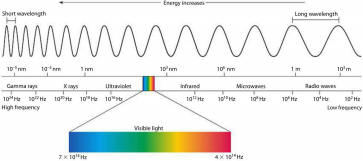o Properties

▪ Wavelength (λ)- peak to peak distance, usually measure in nm

▪ Frequency (ν)- how often a wave cycle passes through a certain point,  measured in hertz (Hz)

**frequency and wavelength are inversely proportional

▪ Amplitude- height from peak to midline of the wave

▪ Intensity- brightness

▪ LIGHT BEHAVES AS BOTH A PARTICLE AND A WAVE

o Calculations

• C = λν, C= 2.998x108 m/s (speed of light in a vacuum)

• E = hν, h= 6.626x10-34 J/s (Planck’s constant)

• E =  hC/λ

Exam 2 Material

• Electron Configuration

o Electron- both a wave and a particle with a defined area of probability o Degenerate- energy equivalency, same n

o Shielding- outer electrons protected by inner layers so they do not feel full effect  of the pull of the positively charged nucleus

o Aufbau principal- order in which electron levels are filled

o Pauli exclusion principle- two different electrons are allowed for each atomic  orbital (↑↓)

o Hund’s Rule- each orbital must be filled once before filling a second time ▪ Right: ↑↓ ↑   ↑

▪ Wrong: ↑ ↑ ↑↓

o Noble gas notation- uses closest noble gas before element

▪ [Noble Gas] Nsl

Atwood, Statler Chem 1210 Fall 2017

• Quantum Numbers

o Each element has its own unique set of quantum numbers

o Principal (n) – overall size and energy of an orbital, distance from nucleus ▪ n= 1, 2, 3, 4, 5, 6, 7

▪ 1: ground state, 2-7: excited state

o Angular (l) – position of electron within the atom, describes shapes

▪ L= 0, 1, 2, 3, 4… 6  l= n-1

▪ 0= s, 1= p, 2= d, 3= f

o Magnetic (ml) – orientation in space, tilt of orbit

▪ ml can range from (– l) to (+ l)

o Spin (ms) – intrinsic spin

▪ ms= +/- 1/2

• Periodic Trends

o Reactivity- how reactive an element is

▪ Highest at bottom of left side (metal) of periodic table and top of right  side (non-metals)

o Ionization Energy- energy required to remove the most loosely bounded electron o Electron Affinity- measure of energy change when an electron is added o Electronegativity- measure of tendency of an atom to attract electrons o Atomic Radius- size of atoms

o Ionic Radius-  size of ions

o HOW TO REMEMBER (on periodic table)

Increasing decreasing

decreasing       I.E.  (ionization energy) increasing A.R. (atomic radius) E.A. (electron affinity) I.R. (ionic radius)

E.N. (electronegativity)

• Writing Ionic Formulas

o Finding number of valence electrons: periodic table column

o Find charge of each ion (also based on column)

o Charges must balance so overall charge of molecule will be 0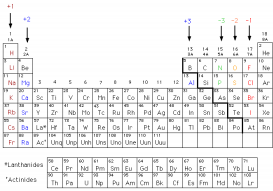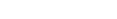CHARGES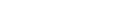VALENCE ELECTRONS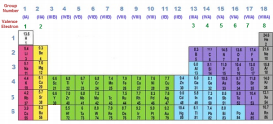• VSEPR

o N – A = S Rule

▪ Only works if it obeys the octet rule

Atwood, Statler Chem 1210 Fall 2017

▪ N- number of electrons needed (8 for each element)

▪ A- available electrons (count valence electrons; add/subtract by charge

for ions)

▪ S- shared (divide by 2 to find number of bonds)

o A – S = number of lone pair electrons

▪ Divide by 2 to find number of pairs

o Elements that don’t typically obey octet rule

▪ B, Al, Ga, P, As, Sb, S, Se, Te, Cl, Br, I, Ar, Kr, Xe

o Electron Groups

▪ Any kind of bond = single group

▪ Lone pair of electrons = single group

▪ Electron groups will try to arrange themselves as far away from each

other as possible

▪ GEOMETRIES

Densities

Electronic Geometry

Possible Molecular Geometries

2

Linear

Linear

3

Trigonal planar

Trigonal planar, bent

4

Tetrahedral

Tetrahedral, trigonal pyramidal, bent

5

Trigonal bipyramidal

Trigonal bipyramidal, seesaw, T-shape,  linear

6

Octahedral

Octahedral, square pyramidal

• Naming Compounds (Nomenclature)

o Binary compounds (2 elements)

▪ Name = metal- nonmetal-ide

▪ Exceptions:

• Oxygen- oxide

• Sulfur- sulfide

• Nitrogen- nitride

• Phosphorous- phosphide

o Binary compounds involving transition metal

▪ Name = metal (charge) nonmetal-ide

▪ Common charged metals:

• Mn, Co, Sn, Fe, Ni, Cu, Pb

o Ionic compounds with polyatomic ion

▪ Name = metal polyatomic ion▪ Polyatomic ions you should know:

Atwood, Statler Chem 1210 Fall 2017

o Hydrated ionic compounds

▪ Name = ionic compound prefix-hydrate

o Two non-metals

▪ Name = prefix- first element prefix-second element ide

o Binary acids

▪ Name = hydro- anion-ic acid

o Oxoacids (depends on which has more O’s)

▪ Name = Polyatomic ion-ous acid

▪ Name = polyatomic ion-ic acid

• Like-Dissolves-Like

o Non-polar solutions will dissolve non-polar molecules

o Polar solution will dissolve polar molecules

o Can determine polarity of a substance based on what dissolves in it/what it  dissolves

• Lewis Structures

o Diagrams that show how a molecule is bonded and where lone pair electrons are  located

o Use VSPER theory in order to draw

o With knowledge of molecular/electronic geometries, we are able to know exact  structure of any given molecule

• Resonance

o When the same molecule can have different structures based on the placement  of particular bonds

o Ex: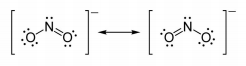• Lattice Energy

o How tight ionic compounds are held together

• Sigma and Pi Bonds

o Sigma (σ)- formed by overlap of orbitals in end-to-end way

o Pi (π)- side-by-side overlap of orbitals

o How many are in each type of bond

▪ Single bond = 1 σ

▪ Double bond = 1 σ 1 π

▪ Triple bond = 1 σ 2 π

• Balancing Equations

o Ensuring that both sides have the same number of the same element o Do so by adding coefficients to products/reactants

o Make sure it’s reduced to the lowest possible ratio

Atwood, Statler Chem 1210 Fall 2017

Exam 3 Material

**refer to “MIDTERM 3 STUDY GUIDE – GENERAL CHEMISTRY I” on my StudySoup page

Post Exam 3 Material

• Redox Reactions

o Definition: a reaction where electrons move from one atom or molecule to  another

o Both reduction and oxidation must be present in order for it to be considered  redox

o Oxidation state- number of electrons that atom already gained or lost when  chemically bonded to make a neutral molecule

o Reduced element- gains electrons, oxidation state lowers

o Oxidized element- loses electrons, oxidation state raises

o Reducing agent- element that is oxidized

o Oxidizing agent- element that is reduced

• Electrolytes

o Strong: dissociate/ionize completely in water, strong acids and strong bases,  soluble salts

o Weak: dissociate/ionize partially in water, weak acids and bases, slightly soluble  salts

o Non-Electrolytes: does not ionize/dissociate, solutions do not conduct electricity • Arrhenius Acids and Bases

o Acids

▪ Strong Acids (7) **remember

• HBr, HI, HCl, HNO3, HClO3, HClO4, H2SO4

▪ Weak Acids

• Carboxylic Acids

• Acetic Acid (CH3COOH)

o Bases

▪ Strong Bases (8) **remember

• LiOH, NaOH, KOH, RbOH, CsOH, CaOH, SrOH, BaOH

▪ Weak Bases

• Metal hydroxides

• Ammonia/amines

• Solubility

o Soluble Salts **MEMORIZE

RULE

EXCEPTION

Group I Salts (Li+, Na+, K+, Rb+, Cs+)

None

Ammonium (NH4+)

None

Nitrates (NO3-)

None

Acetates (CH3COO-)

None

Cl-, Br-, I-

Ag+, Hg22+, Pb 2+

Atwood, Statler Chem 1210 Fall 2017

Sulfates (SO42-)

Ag+, Hg22+, Pb 2+, Ca 2+, Ba 2+, Sr 2+

o Insoluble Salts **MEMORIZE

RULE

EXCEPTION

Carbonate (CO32-)

Group 1 and NH4+

Sulfide (S2-)

Group 1, NH4+, Ca2+, Ba2+, Sr2+

Hydroxides (OH-)

Group 1, NH4+, Ca2+, Ba2+, Sr2+

Phosphate (PO43-)

Group 1 and NH4+

• Net Ionic Eqns.

o What are they

▪ Equations that show only reacting ions and not spectator ions

o What do they show

▪ Show where an actual reaction is taking place

▪ Which elements are spectator ions (cancel out) vs reacting ions (produce  new product)

o How to find them

▪ Write total, balanced chemical equation

▪ Separate any possible substance into its ions (no s, g, or l- only aq); this is  known as the total ionic equation

▪ Cancel out any of the same ions that occur on both sides

▪ Remaining products/reactants are the net ionic equation

• Molarity

o General Equation

▪ M = mol (solute) / L (solution)

o Dilutions

▪ M1V1 = M2V2

▪ Only able to use when diluting with water, doesn’t apply for two different  solutions

• Soln. Stoichiometry

o Using molarity as a conversion factor

o M (molar) = # of mol/1 L

• Acid-Bases Rxns.

o Occur between either a strong acid/weak base reaction –OR- a strong base/weak  acid reaction

Atwood, Statler Chem 1210 Fall 2017

FINAL EXAM STUDY GUIDE – GEN CHEM I

Exam 1 Material

• Unit conversions

o Common ones to know:

▪ 1 in = 2.54 cm

▪ 1 cm3 = 1 mL▪ 1 kg = 2.2 lbs

▪ 1 ton = 2000 lbs

▪ 1 metric ton = 1000 kg

▪ METRIC PREFIXES

▪ Know how to read/write scientific notation

▪ Conversion factors- ratios relating two different units that can be used in  calculations **remember to raise to the same exponent when using in a  problem (ex: (1 m)3 = (100 cm)3 )

o To set up/solve: make sure that the same units are opposite from each other so  they cancel out when entire thing is multiplied

▪ Ex: 12.01 g x 1 mole x 6.022x1023 molecules

12.01 g       1 mole

• Significant Figures: Rules

o All non-zero numbers count as sig figs.

o Zeros in between non-zero numbers count

o Trailing zeros do not count unless there is a decimal point

o Multiplication/division: take answer to the same number of sig figs as the lowest  number of sig figs given in the initial problem

o Addition/subtraction: take answer to the same number of decimal places as the  lowest number of decimal places given in the initial problem

• Protons, Neutrons, and Electrons

o Protons- positively charged, make up center of the atom

o Neutrons- no charge, add mass to the center of the atom

o Electrons- negatively charged, make up the cloud around the outside of the  o Atomic number- identifies which element it is, signified by the number of  protons

Atwood, Statler Chem 1210 Fall 2017

o Atomic weight= number of protons + number of neutrons, can vary if the  element is an isotope (has a different number of neutrons)o Charge- located in top right corner when writing element, usually lines up with  which column the element is in on the periodic table

o All atoms inherently neutral- # of protons = # of electrons

o Ions: charged versions of atoms due to a different number of electrons ▪ Cation- positive, less electrons

▪ Anion- negative, more electrons

o Isotopes: atoms with a different number of neutrons which results in a change of  atomic mass

▪ Percent relative abundance: how frequently an isotope naturally occurs ▪ Atomic mass: weighted average of all natural isotopes of an element

• Scientific Method

o Definition: the process to identify, investigate, and solve problems

o Qualitative observations: descriptive in nature

o Quantitative observations: numbers and measurements

o Hypothesis: educated guess, cause of initial observation

o Experimentation: tests, involves variables and data collection

o Law vs. Theory

▪ Law- conceptual/mathematical statement about what is observed in  nature that has been proven true

▪ Theory- neither proven nor disproven, can link laws to suggest an

underlying theme or principle

• Atomic Theory

o John Dalton (1808) stated that:

▪ Matter is made up of atoms, they are tiny, indivisible particles

▪ All atoms of the same element are identical

▪ Atoms combine in small, whole number ratios to form compounds

▪ Atoms cannot be created nor destroyed

o Law of Conservation of Matter- matter can neither be created nor destroyed,  matter in the universe is fixed and undergoes transformations

▪ Law of Conservation of Mass-Energy (Einstein)- total mass and energy in  the universe is conserved and they can interconvert

o Law of Multiple Proportions- when one element combines with another to form  a compound, the ration of masses of one element to a fixed amount of the  second is a ratio of small, whole numbers

Atwood, Statler Chem 1210 Fall 2017

o Law of Definite Proportions (Constant Composition)- the same compound will be  comprised of constituent elements in the same proportion by mass regardless of  the size of the sample

o J.J. Thompson

▪ Conducted the cathode ray experiment, cathode ray tube between two  charged plates

▪ Ray was attracted to the positive plate, indicated the particles were

negative

▪ Plum Pudding model of the atom: atom is a sphere of a positive charge,  with uniformly embedded negative particles throughout

o Ernest Rutherford

▪ Conducted gold foil experiment, shot alpha particles (positive charge)  through extremely thin piece of gold foil

▪ If plum pudding model was correct (expected), particles would pass

straight through the metal or be slightly deflected

▪ Instead, most of the particles passed straight through, but some were  significantly deflected

▪ Conclusion: particles deflected because of a positive, highly massive

nucleus located in the center of the atom with mostly empty space

remaining

o Robert Millikan

▪ Conducted oil drop experiment,

▪ Determined the charge of an electron to be 1.60x10-19 C

• Mole Conversions

o Amu (atomic mass units)- 1/12th the mass of a C atom, used to measure the  weight of 1 mole of a substance, same as molar mass

▪ 1 mole of any substance contains exactly this many units of the substance  (i.e. molecules, formula units)

o Molar mass- # of grams of a substance per 1 mole, located on the periodic table,  add up all the molar masses of elements in a compound to find it’s molar mass o Set up and solve the same way as for unit conversions

▪ g/mol

▪ molecules/mol

▪ mol element/1 mol compound

• Atomic Spectrum

o Bohr Model of the Atom

▪ Angular momentum of electrons and their radii were quantized, travel in  fixed orbits, and each orbit has energy associated with it

o Electron transitions

▪ Each increasing level has a lower distance between it and the previous  one (first energy jump is the largest, then get progressively smaller)

▪ The larger the distance, the more energy it requires to transition7

Atwood, Statler Chem 1210 Fall 2017

▪ ΔEn = -2.178x1018 (1/n2f – 1/n2i)

• Light

o Electromagnetic Spectrumo Properties

▪ Wavelength (λ)- peak to peak distance, usually measure in nm

▪ Frequency (ν)- how often a wave cycle passes through a certain point,  measured in hertz (Hz)

**frequency and wavelength are inversely proportional

▪ Amplitude- height from peak to midline of the wave

▪ Intensity- brightness

▪ LIGHT BEHAVES AS BOTH A PARTICLE AND A WAVE

o Calculations

• C = λν, C= 2.998x108 m/s (speed of light in a vacuum)

• E = hν, h= 6.626x10-34 J/s (Planck’s constant)

• E =  hC/λ

Exam 2 Material

• Electron Configuration

o Electron- both a wave and a particle with a defined area of probability o Degenerate- energy equivalency, same n

o Shielding- outer electrons protected by inner layers so they do not feel full effect  of the pull of the positively charged nucleus

o Aufbau principal- order in which electron levels are filled

o Pauli exclusion principle- two different electrons are allowed for each atomic  orbital (↑↓)

o Hund’s Rule- each orbital must be filled once before filling a second time ▪ Right: ↑↓ ↑   ↑

▪ Wrong: ↑ ↑ ↑↓

o Noble gas notation- uses closest noble gas before element

▪ [Noble Gas] Nsl

Atwood, Statler Chem 1210 Fall 2017

• Quantum Numbers

o Each element has its own unique set of quantum numbers

o Principal (n) – overall size and energy of an orbital, distance from nucleus ▪ n= 1, 2, 3, 4, 5, 6, 7

▪ 1: ground state, 2-7: excited state

o Angular (l) – position of electron within the atom, describes shapes

▪ L= 0, 1, 2, 3, 4… 6  l= n-1

▪ 0= s, 1= p, 2= d, 3= f

o Magnetic (ml) – orientation in space, tilt of orbit

▪ ml can range from (– l) to (+ l)

o Spin (ms) – intrinsic spin

▪ ms= +/- 1/2

• Periodic Trends

o Reactivity- how reactive an element is

▪ Highest at bottom of left side (metal) of periodic table and top of right  side (non-metals)

o Ionization Energy- energy required to remove the most loosely bounded electron o Electron Affinity- measure of energy change when an electron is added o Electronegativity- measure of tendency of an atom to attract electrons o Atomic Radius- size of atoms

o Ionic Radius-  size of ions

o HOW TO REMEMBER (on periodic table)

Increasing decreasing

decreasing       I.E.  (ionization energy) increasing A.R. (atomic radius) E.A. (electron affinity) I.R. (ionic radius)

E.N. (electronegativity)

• Writing Ionic Formulas

o Finding number of valence electrons: periodic table column

o Find charge of each ion (also based on column)

o Charges must balance so overall charge of molecule will be 0CHARGES

VALENCE ELECTRONS• VSEPR

o N – A = S Rule

▪ Only works if it obeys the octet rule

Atwood, Statler Chem 1210 Fall 2017

▪ N- number of electrons needed (8 for each element)

▪ A- available electrons (count valence electrons; add/subtract by charge

for ions)

▪ S- shared (divide by 2 to find number of bonds)

o A – S = number of lone pair electrons

▪ Divide by 2 to find number of pairs

o Elements that don’t typically obey octet rule

▪ B, Al, Ga, P, As, Sb, S, Se, Te, Cl, Br, I, Ar, Kr, Xe

o Electron Groups

▪ Any kind of bond = single group

▪ Lone pair of electrons = single group

▪ Electron groups will try to arrange themselves as far away from each

other as possible

▪ GEOMETRIES

Densities

Electronic Geometry

Possible Molecular Geometries

2

Linear

Linear

3

Trigonal planar

Trigonal planar, bent

4

Tetrahedral

Tetrahedral, trigonal pyramidal, bent

5

Trigonal bipyramidal

Trigonal bipyramidal, seesaw, T-shape,  linear

6

Octahedral

Octahedral, square pyramidal

• Naming Compounds (Nomenclature)

o Binary compounds (2 elements)

▪ Name = metal- nonmetal-ide

▪ Exceptions:

• Oxygen- oxide

• Sulfur- sulfide

• Nitrogen- nitride

• Phosphorous- phosphide

o Binary compounds involving transition metal

▪ Name = metal (charge) nonmetal-ide

▪ Common charged metals:

• Mn, Co, Sn, Fe, Ni, Cu, Pb

o Ionic compounds with polyatomic ion

▪ Name = metal polyatomic ion

▪ Polyatomic ions you should know:

Atwood, Statler Chem 1210 Fall 2017

o Hydrated ionic compounds

▪ Name = ionic compound prefix-hydrate

o Two non-metals

▪ Name = prefix- first element prefix-second element ide

o Binary acids

▪ Name = hydro- anion-ic acid

o Oxoacids (depends on which has more O’s)

▪ Name = Polyatomic ion-ous acid

▪ Name = polyatomic ion-ic acid

• Like-Dissolves-Like

o Non-polar solutions will dissolve non-polar molecules

o Polar solution will dissolve polar molecules

o Can determine polarity of a substance based on what dissolves in it/what it  dissolves

• Lewis Structures

o Diagrams that show how a molecule is bonded and where lone pair electrons are  located

o Use VSPER theory in order to draw

o With knowledge of molecular/electronic geometries, we are able to know exact  structure of any given molecule

• Resonance

o When the same molecule can have different structures based on the placement  of particular bonds

o Ex:

• Lattice Energy

o How tight ionic compounds are held together

• Sigma and Pi Bonds

o Sigma (σ)- formed by overlap of orbitals in end-to-end way

o Pi (π)- side-by-side overlap of orbitals

o How many are in each type of bond

▪ Single bond = 1 σ

▪ Double bond = 1 σ 1 π

▪ Triple bond = 1 σ 2 π

• Balancing Equations

o Ensuring that both sides have the same number of the same element o Do so by adding coefficients to products/reactants

o Make sure it’s reduced to the lowest possible ratio

Atwood, Statler Chem 1210 Fall 2017

Exam 3 Material

**refer to “MIDTERM 3 STUDY GUIDE – GENERAL CHEMISTRY I” on my StudySoup page

Post Exam 3 Material

• Redox Reactions

o Definition: a reaction where electrons move from one atom or molecule to  another

o Both reduction and oxidation must be present in order for it to be considered  redox

o Oxidation state- number of electrons that atom already gained or lost when  chemically bonded to make a neutral molecule

o Reduced element- gains electrons, oxidation state lowers

o Oxidized element- loses electrons, oxidation state raises

o Reducing agent- element that is oxidized

o Oxidizing agent- element that is reduced

• Electrolytes

o Strong: dissociate/ionize completely in water, strong acids and strong bases,  soluble salts

o Weak: dissociate/ionize partially in water, weak acids and bases, slightly soluble  salts

o Non-Electrolytes: does not ionize/dissociate, solutions do not conduct electricity • Arrhenius Acids and Bases

o Acids

▪ Strong Acids (7) **remember

• HBr, HI, HCl, HNO3, HClO3, HClO4, H2SO4

▪ Weak Acids

• Carboxylic Acids

• Acetic Acid (CH3COOH)

o Bases

▪ Strong Bases (8) **remember

• LiOH, NaOH, KOH, RbOH, CsOH, CaOH, SrOH, BaOH

▪ Weak Bases

• Metal hydroxides

• Ammonia/amines

• Solubility

o Soluble Salts **MEMORIZE

RULE

EXCEPTION

Group I Salts (Li+, Na+, K+, Rb+, Cs+)

None

Ammonium (NH4+)

None

Nitrates (NO3-)

None

Acetates (CH3COO-)

None

Cl-, Br-, I-

Ag+, Hg22+, Pb 2+

Atwood, Statler Chem 1210 Fall 2017

Sulfates (SO42-)

Ag+, Hg22+, Pb 2+, Ca 2+, Ba 2+, Sr 2+

o Insoluble Salts **MEMORIZE

RULE

EXCEPTION

Carbonate (CO32-)

Group 1 and NH4+

Sulfide (S2-)

Group 1, NH4+, Ca2+, Ba2+, Sr2+

Hydroxides (OH-)

Group 1, NH4+, Ca2+, Ba2+, Sr2+

Phosphate (PO43-)

Group 1 and NH4+

• Net Ionic Eqns.

o What are they

▪ Equations that show only reacting ions and not spectator ions

o What do they show

▪ Show where an actual reaction is taking place

▪ Which elements are spectator ions (cancel out) vs reacting ions (produce  new product)

o How to find them

▪ Write total, balanced chemical equation

▪ Separate any possible substance into its ions (no s, g, or l- only aq); this is  known as the total ionic equation

▪ Cancel out any of the same ions that occur on both sides

▪ Remaining products/reactants are the net ionic equation

• Molarity

o General Equation

▪ M = mol (solute) / L (solution)

o Dilutions

▪ M1V1 = M2V2

▪ Only able to use when diluting with water, doesn’t apply for two different  solutions

• Soln. Stoichiometry

o Using molarity as a conversion factor

o M (molar) = # of mol/1 L

• Acid-Bases Rxns.

o Occur between either a strong acid/weak base reaction –OR- a strong base/weak  acid reaction

Page ExpiredIt looks like your free minutes have expired! Lucky for you we have all the content you need, just sign up here